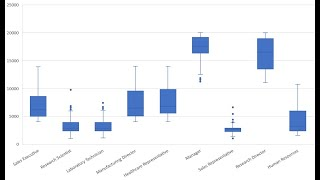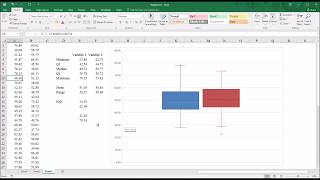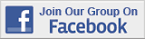Excel Tips & Tutorials:
Graphing Relationships and Describing Patterns
 Suggest a Link Alphabetize Page Printer-Friendly List
Watch and Learn
Watch and Learn
Watch and Learn
Watch and Learn
 How to Create a BoxplotComparing numeric values across multiple categories (2:02).How to Make a Line GraphShow you how to create and edit a line chart (6:07).Creating a BoxplotTutorial from Todd Grande (12:03).How to Create 100% Stacked Column ChartChart option for qualitative dependent variable (4:58).How to Create a BoxplotComparing numeric values across multiple categories (2:02).How to Make a Line GraphShow you how to create and edit a line chart (6:07).Creating a BoxplotTutorial from Todd Grande (12:03).How to Create a BoxplotComparing numeric values across multiple categories (2:02).How to Make a Line GraphShow you how to create and edit a line chart (6:07).How to Create a BoxplotComparing numeric values across multiple categories (2:02).Over 18,000 Members!